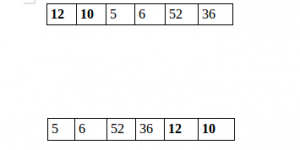Related Articles

# Java Program to Split the array and add the first part to the end

• Last Updated : 05 Dec, 2018

There is a given an array and split it from a specified position, and move the first part of array add to the end.Examples:

```Input : arr[] = {12, 10, 5, 6, 52, 36}
k = 2
Output : arr[] = {5, 6, 52, 36, 12, 10}
Explanation : Split from index 2 and first
part {12, 10} add to the end .

Input : arr[] = {3, 1, 2}
k = 1
Output : arr[] = {1, 2, 3}
Explanation : Split from index 1 and first
```

## Recommended: Please try your approach on {IDE} first, before moving on to the solution.

 `// Java program to split array and move first``// part to end.`` ` `import` `java.util.*;``import` `java.lang.*;``class` `GFG {``    ``public` `static` `void` `splitArr(``int` `arr[], ``int` `n, ``int` `k)``    ``{``        ``for` `(``int` `i = ``0``; i < k; i++) {`` ` `            ``// Rotate array by 1.``            ``int` `x = arr[``0``];``            ``for` `(``int` `j = ``0``; j < n - ``1``; ++j)``                ``arr[j] = arr[j + ``1``];``            ``arr[n - ``1``] = x;``        ``}``    ``}`` ` `    ``// Driver code``    ``public` `static` `void` `main(String[] args)``    ``{``        ``int` `arr[] = { ``12``, ``10``, ``5``, ``6``, ``52``, ``36` `};``        ``int` `n = arr.length;``        ``int` `position = ``2``;`` ` `        ``splitArr(arr, ``6``, position);`` ` `        ``for` `(``int` `i = ``0``; i < n; ++i)``            ``System.out.print(arr[i] + ``" "``);``    ``}``}`` ` `// Code Contributed by Mohit Gupta_OMG <(0_o)>`
Output:
```5 6 52 36 12 10
```

Please refer complete article on Split the array and add the first part to the end for more details!

My Personal Notes arrow_drop_up